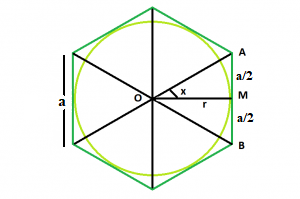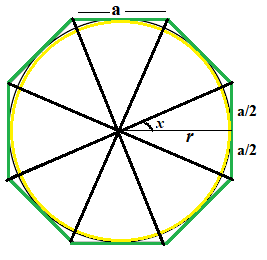# Area of largest Circle inscribe in N-sided Regular polygon

• Last Updated : 19 Jan, 2022

Given a regular polygon of N sides with side length a. The task is to find the area of the Circle which inscribed in the polygon.
Note : This problem is mixed version of This and This
Examples:

```Input: N = 6, a = 4
Output: 37.6801
Explanation:``````In this, the polygon have 6 faces
and as we see in fig.1 we clearly see
that the angle  x  is 30 degree
so the radius of circle will be ( a / (2  * tan(30)))
Therefore, r = a√3/2

Input: N = 8, a = 8
Output: 292.81
Explanation:``````In this, the polygon have 8 faces
and as we see in fig.2 we clearly see
that the angle  x  is 22.5 degree
so the radius of circle will be ( a / (2  * tan(22.5)))
Therefore, r = a/0.828```

Approach: In the figure above, we see the polygon can be divided into N equal triangles. Looking into one of the triangles, we see that the whole angle at the center can be divided into = 360/N
So, angle x = 180/n
Now, tan(x) = (a / 2) * r
So, r = a / ( 2 * tan(x))
So, Area of the Inscribed Circle is,

` A = Πr² = Π * (a / (2 * tan(x))) * (a / (2*tan(x)))`

Below is the implementation of the above approach:

## C++

 `// C++ Program to find the area of a circle in``// inscribed in polygon` `#include ``using` `namespace` `std;` `// Function to find the area``// of a circle``float` `InscribedCircleArea(``float` `n, ``float` `a)``{``    ``// Side and side length cannot be negative``    ``if` `(a < 0 && n < 0)``        ``return` `-1;` `    ``// degree converted to radians``    ``float` `r = a / (2 * ``tan``((180 / n) * 3.14159 / 180));` `    ``// area of circle``    ``float` `Area = (3.14) * (r) * (r);` `    ``return` `Area;``}` `// Driver code``int` `main()``{` `    ``// no.  of sides``    ``float` `n = 6;` `    ``// side length``    ``float` `a = 4;` `    ``cout << InscribedCircleArea(n, a) << endl;` `    ``return` `0;``}`

## Java

 `// Java Program to find the area of a circle``// inscribed in a polygon``import` `java.io.*;` `class` `GFG {` `    ``// Function to find the area``    ``// of a regular polygon``    ``static` `float` `InscribedCircleArea(``float` `n, ``float` `a)``    ``{``        ``// Side and side length cannot be negative``        ``if` `(a < ``0` `&& n < ``0``)``            ``return` `-``1``;` `        ``// degree converted to radians``        ``float` `r = a / (``float``)(``2` `* Math.tan((``180` `/ n) * ``3.14159` `/ ``180``));` `        ``// area of circle``        ``float` `Area = (``float``)(``3.14``) * (r) * (r);` `        ``return` `Area;``    ``}` `    ``// Driver code` `    ``public` `static` `void` `main(String[] args)``    ``{` `        ``// no.  of sides``        ``float` `n = ``6``;` `        ``// side length``        ``float` `a = ``4``;` `        ``System.out.println(InscribedCircleArea(n, a));``    ``}``}`

## Python3

 `# Python 3 Program to find the area``# of a circle inscribed``# in a polygon``from` `math ``import` `tan` `# Function to find the area of a``# circle``def` `InscribedCircleArea(n, a):``    ``# Side and side length cannot``    ``# be negative``    ``if` `(a < ``0` `and` `n < ``0``):``        ``return` `-``1` `    ``# degree converted to radians``    ``r ``=` `a``/``(``2` `*` `tan((``180` `/` `n) ``*` `3.14159` `/` `180``));` `    ``# area of circle``    ``Area ``=` `3.14` `*` `r ``*` `r` `    ``return` `Area` `# Driver code``if` `__name__ ``=``=` `'__main__'``:``    ``a ``=` `4``    ``n ``=` `6` `    ``print``(``'{0:.6}'``.``format``(InscribedCircleArea(n, a)))` `# This code is contributed by``# Chandan Agrawal`

## C#

 `// C# Program to find the area of a circle``// inscribed in a polygon``using` `System;` `class` `GFG``{` `// Function to find the area``// of a regular polygon``static` `float` `InscribedCircleArea(``float` `n, ``float` `a)``{``    ``// Side and side length cannot be negative``    ``if` `(a < 0 && n < 0)``        ``return` `-1;` `    ``// degree converted to radians``    ``float` `r = a / (``float``)(2 * Math.Tan((180 / n) *``                                 ``3.14159 / 180));` `    ``// area of circle``    ``float` `Area = (``float``)(3.14) * (r) * (r);` `    ``return` `Area;``}` `// Driver code``public` `static` `void` `Main()``{` `    ``// no. of sides``    ``float` `n = 6;` `    ``// side length``    ``float` `a = 4;` `    ``Console.WriteLine(InscribedCircleArea(n, a));``}``}` `// This code is contributed by Ryuga`

## PHP

 ``

## Javascript

 ``
Output:
`37.6801`

My Personal Notes arrow_drop_up